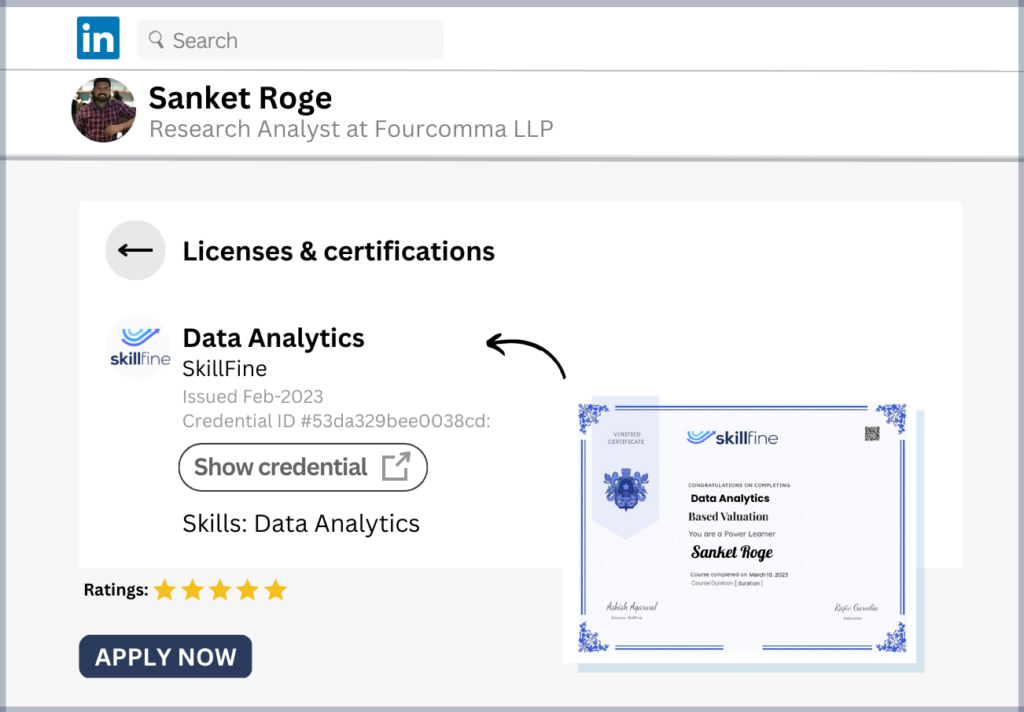# The Filter Function in Data Analytics: Examples and Use Cases## The Filter Function in Data Analytics: Examples and Use Cases

The Filter function is a newly introduced function in Microsoft Excel 365. It is not available in earlier versions of excel. In earlier versions, Filtering of large data sets was done manually. With the new Filter function, filtering of data can now be done dynamically. This function is very useful for data cleaning as well as analysis.

Filter function can be used to filter a range of data based on defined criteria(s).

## Filter function syntax

The filter function has 3 arguments.

=Filter(Array,Include,[if_empty])

The first 2 arguments are compulsory. The third one is optional.

Array is the range we want to filter.

Include is the column or row range to be filtered. We also define the criteria based on which the data has to be filtered.

If Empty is the data to be shown in case the filtered data is not found in the data set.

Let us understand the filter function with the help of a few examples.

Click to learn top 10 financial functions in Excel

### Example 1 – Filtering customer names by region

Here is a list of customers by regions and revenues during a particular period. We want to filter the data by regions.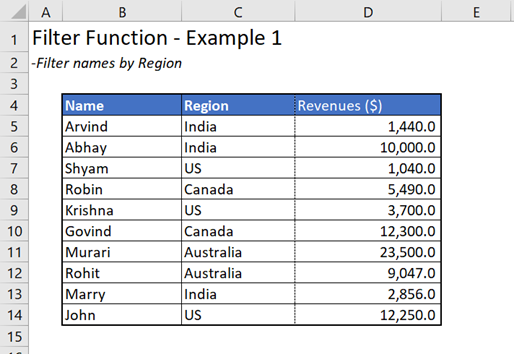To filter the data by region. Type

=Filter(B5:D14,C5:C14=”India”)

B5:B14 is the array

C5:C14 is the column range for which data has to be filtered. We can filter the data by entering the name of the country in Inverted commas. For Example, “India” as mentioned above.

The third argument is optional. We can leave it blank.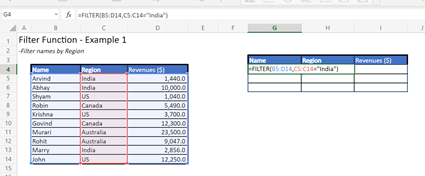When we press enter. We get the data filtered for India.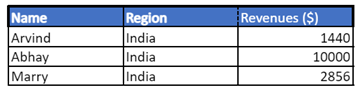We can also use the data validation feature in excel to select the country for which we want to filter the data. In that case, instead of typing the name of the country, we can link the column to the data validation list.

Read more how can you do data automation using excel

### Example 2 – Filtering customers by revenues

We have the same data set used in our previous example. We want to filter the customers whose revenues is greater than \$5,000/-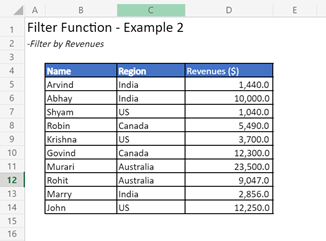To filter the name of customers with \$5,000+ revenues. Type

=Filter(B5:D14,D5:D14>5000)

B5:B14 is the array

D5:D14 is the column range for which data has to be filtered. We can filter the data by entering the criteria. For Example, >5000 as mentioned above.

The third argument is optional. We can leave it blank.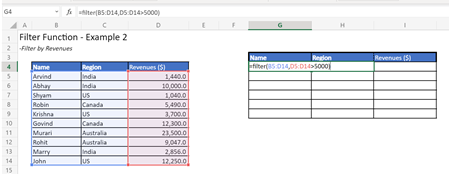Press Enter

You get the name of the customers with Revenues greater than \$5,000.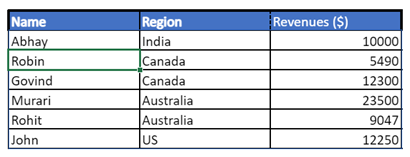### Example 3 – Filtering data with multiple conditions

In the given data set, we want to filter the name of customers in US with revenues less than \$5,000.

There are two conditions here.

• The customer is in US
• Revenues are less than \$5,000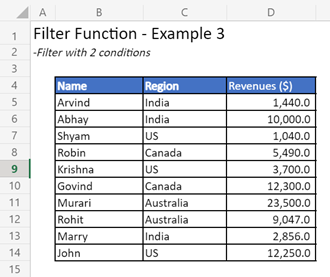To filter the data based on these two criteria’s, Type

=Filter(B5:D14,(C5:C14=“US”)*(D5:D14<5000))

B5:B14 is the array

The second input is divided into two parts.

• (C5:C14=“US”) to filter the data for customers in US.
• (D5:D14<5000) to filter the customers in US with revenues less than \$5,000.
• To join the two conditions we have the asterisk (*) sign in between.

The third argument is optional. We can leave it blank.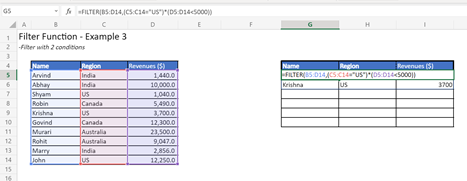Press Enter.

You get the filtered data.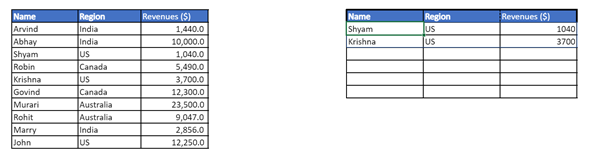There are two customers in US with revenues less than \$5,000.

Get to know How to Transpose Data in Excel

### Example 4 – Sorting and Filtering customers by revenues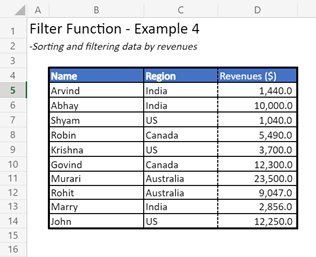In this example we will use the Sort Function and Filter function together.

We want to filter the data for customers whose revenues is greater than \$5,000/-. The data needs to be arranged in alphabetical order.

Like we did in Example 2, to filter the data, Type

=Filter(B5:D14,D5:D14>5000)

B5:B14 is the array

D5:D14 is the column range for which data has to be filtered. We can filter the data by entering the criteria. For Example, >5000 as mentioned above.

The third argument is optional. We can leave it blank.

When we press enter, we get the filtered data.

Now to sort the data in alphabetical order, we will use the sort function.

Type

=Sort(Filter(B5:D14,D5:D14>5000))

Sort function has other arguments as well, but since we have to get this data in alphabetical order, we don’t need any further inputs.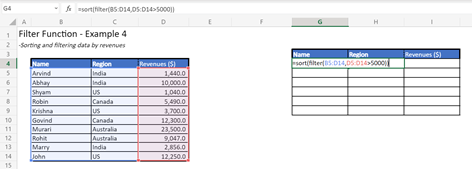Press Enter.

We get the list of customers whose revenue is greater than \$5,000/- sorted in alphabetical order.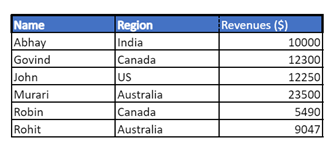This is how we can use the Filter function. It can be used for cleaning/ analys

Learn Excel Shortcuts

Learn XLookup

Let us know in case you have any questions, by posting in the comment section below.

Happy learning.